Author: Oscar Cronquist Article last updated on October 15, 2019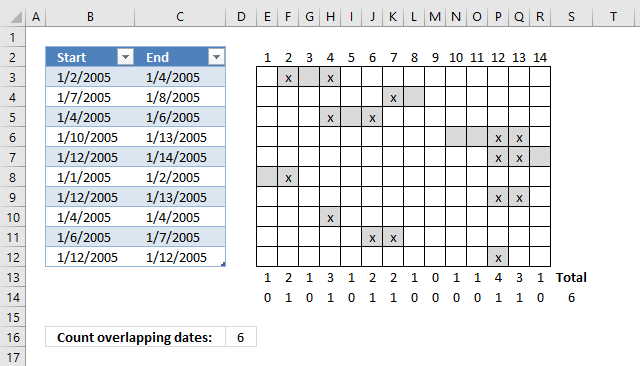This post demonstrates a formula in cell D16 that counts overlapping dates across multiple date ranges. The date ranges are specified in the Excel Table column Start and End (cell range B3:B12 and C3:C12), see image above.

To the right is a part of a calendar with date 1 to 14, the gray cells are days based on the corresponding date range. The x's are dates that overlap another date.

Row 13 contains the sum of these gray days column-wise, row 14 contains 1 or 0 (zero) indicating if the date contains a date range that overlaps another date range. Cell S14 contains a total of these overlapping dates.  This is only demonstrated to show that the calculation is in fact correct.

Thanks a tonne, Oscar. It took me about 8 hours to work through this formula piece by piece, play with it, and come to grips with its basics. Your example was clear and very useful, and this has allowed me to do big, very useful data analysis for the company that employs me. It applies to thousands of people. You're an unsung hero.

Actually just realized that I really need what you said you'd "save for a future post" (actually total number of overlapping dates). I guess I'll try to figure that out. Still... couldn't have gotten this close without you.

Thanks NC, here comes that "future" post. Array formula in cell D16:

=SUM((MMULT(TRANSPOSE(Table1[Start]^0), (TRANSPOSE(MIN(Table1[Start])+ROW(B1:INDEX(\$B:\$B, MAX(Table1[End])-MIN(Table1[Start])+1))-1)>=Table1[Start])*((TRANSPOSE(MIN(Table1[Start])+ROW(B1:INDEX(\$B:\$B, MAX(Table1[End])-MIN(Table1[Start])+1))-1))<=Table1[End]))>1)*1)

To enter an array formula you copy above formula and paste to a cell. Press and hold CTRL and Shift simultaneoulsy, then press Enter once. Release all keys.

The formula is now surrounded with curly brackets, don't enter these characters yourself, they appear automatically. {=formula}

### Explaining formula in cell D16

#### Step 1 - Create array

The power of sign ^ converts all dates in Table column Start to 1, you can also use the POWER function, however, to keep the formula as small as possible I use ^.

TRANSPOSE(Table1[Start]^0)

becomes

TRANSPOSE({38354; 38359; 38356; 38362; 38364; 38353; 38364; 38356; 38358; 38364}^0)

becomes

TRANSPOSE({1; 1; 1; 1; 1; 1; 1; 1; 1; 1})

The TRANSPOSE function converts a vertical range to a horizontal range, or vice versa.

TRANSPOSE({1; 1; 1; 1; 1; 1; 1; 1; 1; 1})

returns

{1, 1, 1, 1, 1, 1, 1, 1, 1, 1}

This array is needed in the first argument in the MMULT function.

#### Step 2 - Create an array containing dates

This step calculates the dates needed based on the earliest and latest date in columns Table1[Start] and Table1[End].

(TRANSPOSE(MIN(Table1[Start])+ROW(B1:INDEX(\$B:\$B,MAX(Table1[End])-MIN(Table1[Start])+1))-1)

becomes

(TRANSPOSE(MIN({38354; 38359; 38356; 38362; 38364; 38353; 38364; 38356; 38358; 38364})+ROW(B1:INDEX(\$B:\$B,MAX(Table1[End])-MIN(Table1[Start])+1))-1)

becomes

(TRANSPOSE(38353+ROW(B1:INDEX(\$B:\$B,MAX(Table1[End])-MIN(Table1[Start])+1))-1)

becomes

(TRANSPOSE(38353+ROW(B1:INDEX(\$B:\$B,MAX({38356; 38360; 38358; 38365; 38366; 38354; 38365; 38356; 38359; 38364})-MIN(Table1[Start])+1))-1)

becomes

(TRANSPOSE(38353+ROW(B1:INDEX(\$B:\$B,38366-MIN(Table1[Start])+1))-1)

becomes

(TRANSPOSE(38353+ROW(B1:INDEX(\$B:\$B,38366-38353+1)))-1)

becomes

(TRANSPOSE(38353+ROW(B1:INDEX(\$B:\$B,13+1)))-1)

becomes

(TRANSPOSE(38353+ROW(B1:INDEX(\$B:\$B,14)))-1)

becomes

(TRANSPOSE(38353+ROW(B1:\$B14))-1)

becomes

(TRANSPOSE(38353+{1; 2; 3; 4; 5; 6; 7; 8; 9; 10; 11; 12; 13; 14})-1)

becomes

(TRANSPOSE({38354; 38355; 38356; 38357; 38358; 38359; 38360; 38361; 38362; 38363; 38364; 38365; 38366; 38367})-1)

becomes

(TRANSPOSE(38353+{0; 1; 2; 3; 4; 5; 6; 7; 8; 9; 10; 11; 12; 13})

and returns

{38353, 38354, 38355, 38356, 38357, 38358, 38359, 38360, 38361, 38362, 38363, 38364, 38365, 38366}

#### Step 3 - Check which dates are later or equal to dates in Table column Start

(TRANSPOSE(MIN(Table1[Start])+ROW(B1:INDEX(\$B:\$B,MAX(Table1[End])-MIN(Table1[Start])+1))-1)>=Table1[Start]

becomes

{38353, 38354, 38355, 38356, 38357, 38358, 38359, 38360, 38361, 38362, 38363, 38364, 38365, 38366}>=Table1[Start]

becomes

{38353, 38354, 38355, 38356, 38357, 38358, 38359, 38360, 38361, 38362, 38363, 38364, 38365, 38366}>={38354; 38359; 38356; 38362; 38364; 38353; 38364; 38356; 38358; 38364}

and returns this array: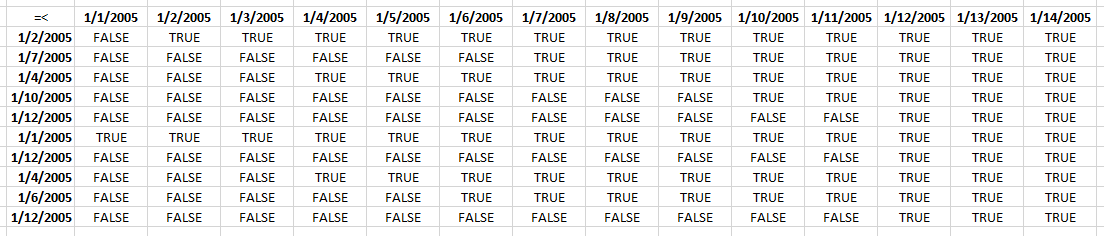I have added the dates horizontally and vertically to make this picture more easy to understand.

#### Step 4 - Repeat step 2 and 3 with Table column End

((TRANSPOSE(MIN(Table1[Start])+ROW(B1:INDEX(\$B:\$B,MAX(Table1[End])-MIN(Table1[Start])+1))-1))<=Table1[End]))>1)

returns this array: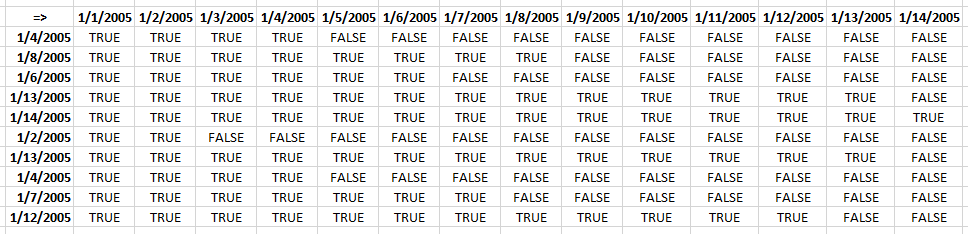#### Step 5 - Multiply arrays

(TRANSPOSE(MIN(Table1[Start])+ROW(B1:INDEX(\$B:\$B, MAX(Table1[End])-MIN(Table1[Start])+1))-1)>=Table1[Start])*((TRANSPOSE(MIN(Table1[Start])+ROW(B1:INDEX(\$B:\$B, MAX(Table1[End])-MIN(Table1[Start])+1))-1))<=Table1[End])

returns this array: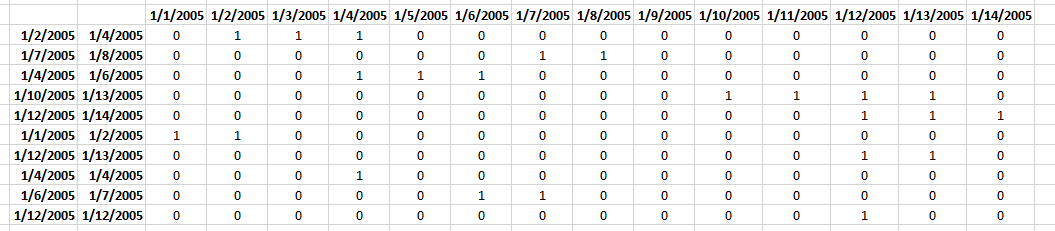#### Step 6 - Sum values column-wise

MMULT(TRANSPOSE(Table1[Start]^0), (TRANSPOSE(MIN(Table1[Start])+ROW(B1:INDEX(\$B:\$B, MAX(Table1[End])-MIN(Table1[Start])+1))-1)>=Table1[Start])*((TRANSPOSE(MIN(Table1[Start])+ROW(B1:INDEX(\$B:\$B, MAX(Table1[End])-MIN(Table1[Start])+1))-1))<=Table1[End]))

returns

{1, 2, 1, 3, 1, 2, 2, 1, 0, 1, 1, 4, 3, 1}

#### Step 7 - Check if value in array is larger than 1

MMULT(TRANSPOSE(Table1[Start]^0), (TRANSPOSE(MIN(Table1[Start])+ROW(B1:INDEX(\$B:\$B, MAX(Table1[End])-MIN(Table1[Start])+1))-1)>=Table1[Start])*((TRANSPOSE(MIN(Table1[Start])+ROW(B1:INDEX(\$B:\$B, MAX(Table1[End])-MIN(Table1[Start])+1))-1))<=Table1[End]))>1

becomes

{1, 2, 1, 3, 1, 2, 2, 1, 0, 1, 1, 4, 3, 1}>1

and returns

{FALSE, TRUE, FALSE, TRUE, FALSE, TRUE, TRUE, FALSE, FALSE, FALSE, FALSE, TRUE, TRUE, FALSE}

#### Step 8 - Convert boolean values to their numerical equivalents

(MMULT(TRANSPOSE(Table1[Start]^0), (TRANSPOSE(MIN(Table1[Start])+ROW(B1:INDEX(\$B:\$B, MAX(Table1[End])-MIN(Table1[Start])+1))-1)>=Table1[Start])*((TRANSPOSE(MIN(Table1[Start])+ROW(B1:INDEX(\$B:\$B, MAX(Table1[End])-MIN(Table1[Start])+1))-1))<=Table1[End]))>1)*1

becomes

{FALSE, TRUE, FALSE, TRUE, FALSE, TRUE, TRUE, FALSE, FALSE, FALSE, FALSE, TRUE, TRUE, FALSE}*1

and returns

{0, 1, 0, 1, 0, 1, 1, 0, 0, 0, 0, 1, 1, 0}

#### Step 9 - Sum overlapping dates

SUM((MMULT(TRANSPOSE(Table1[Start]^0), (TRANSPOSE(MIN(Table1[Start])+ROW(B1:INDEX(\$B:\$B, MAX(Table1[End])-MIN(Table1[Start])+1))-1)>=Table1[Start])*((TRANSPOSE(MIN(Table1[Start])+ROW(B1:INDEX(\$B:\$B, MAX(Table1[End])-MIN(Table1[Start])+1))-1))<=Table1[End]))>1)*1)

becomes

SUM({0, 1, 0, 1, 0, 1, 1, 0, 0, 0, 0, 1, 1, 0})

and returns 6 in cell D16.

### Get the Excel fileCount-overlapping-days-in-multiple-date-ranges-part2.xlsx

### Count overlapping days across multiple date ranges with condition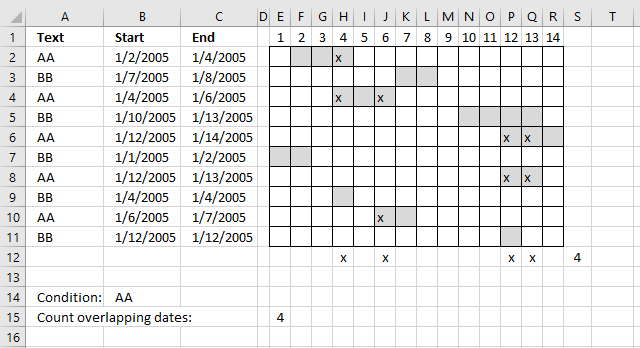This formula takes into account the condition specified in cell E15 as well, the formula counts overlapping dates based on date ranges that has a cell in column A which is equal to the condition in cell B14.

Formula in cell E15:

=SUM((MMULT(TRANSPOSE(\$B\$2:\$B\$11^0), (TRANSPOSE(MIN(\$B\$2:\$B\$11)+ROW(B1:INDEX(\$B:\$B, MAX(\$C\$2:\$C\$11)-MIN(\$B\$2:\$B\$11)+1))-1)>=\$B\$2:\$B\$11)*((TRANSPOSE(MIN(\$B\$2:\$B\$11)+ROW(B1:INDEX(\$B:\$B, MAX(\$C\$2:\$C\$11)-MIN(\$B\$2:\$B\$11)+1))-1))<=\$C\$2:\$C\$11)*(\$A\$2:\$A\$11=\$B\$14))>1)*1)

The only difference with the formula above is this part:

(\$A\$2:\$A\$11=\$B\$14)

It is a logical expression that returns an array containing TRUE or FALSE if equal to value in cell B14.

### Get the Excel fileCount-overlapping-days-in-multiple-date-ranges-with-condition.xlsx